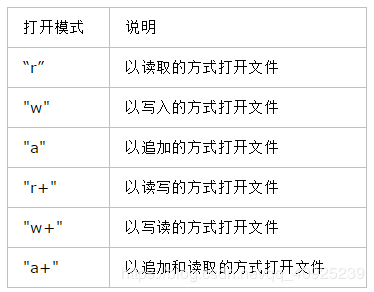﻿ - 代码学院 www.codexy.cn

# python游戏开发：pygame中的IO、数据

1.输出

python一次可以打印多个变量，只要用一个逗号将每个变量隔开就可以了。比如：

` A = 123 B = "ABC" C = 456 D = "DEF" print(A,B,C,D)`

` import sys print(sys.copyright) print(sys.platform) print(sys.version)`

2.获取用户的输入：

` name = raw_input("Hi! what's your name?") print(name)`

3.异常处理

` `s = input("Enter a number: ") try: number = float(s) except: number = 0 answer = number * number print(number,"*",number,"=",answer)``

1.操作文本文件：` `#调用open函数打开一个文件 file =open("data.txt","r") #在完成操作后要将文件关闭 file.close()``

` file = open("data2.txt", "w") file.write("abcdefg\n") file.close()`

` `text_lines = [ "abc\n", "efg\n", "hij\n" ] file = open("data.txt", "w") file.writelines(text_lines) file.close()``

` file = open("data.txt", "r")`

` char = file.read(10) print (char) #这段代码会从文件中的当前指针位置读取10个字符 #像这样重复的调用，将继续从该文件读取更多的字符 #并且向前推进指针的位置`

` file = open("data.txt", "r") all_data = file.readlines() print(all_data) file.close()`

` print("Lines: ", len(all_data)) for line in all_data: print(line.strip())`

String.strip()方法会删去行末的换行字符。

2.操作二进制文件

 打开模式 说明 “rb” 以读取的方式打开二进制文件 "wb" 以写入的方式打开二进制文件 "ab" 以追加的方式打开二进制文件 "rb+" 以读取写入的方式打开二进制文件 "wb+" 以写入读取的方式打开二进制文件 "ab+" 以追加和读取的方式打开二进制文件

1.写入二进制文件：

` `import struct file = open("bin.dat", "wb") for n in range(100): data = struct.pack('i', n) file.write(data) file.close() #这段代码是将100个整数写入到文件中``

2.读取二进制文件

` `file = open("bin.dat", "rb") size = struct.calcsize("i") bytes_read = file.read(size) while bytes_read: value = struct.unpack("i", bytes_read) value = value print(value, end=" ") bytes_read = file.read(size) file.close()``
` struct.calcsize("i")可以计算出一个int类型的大小，以便让struct.unpack知道应该读取多少个字节的数据。`